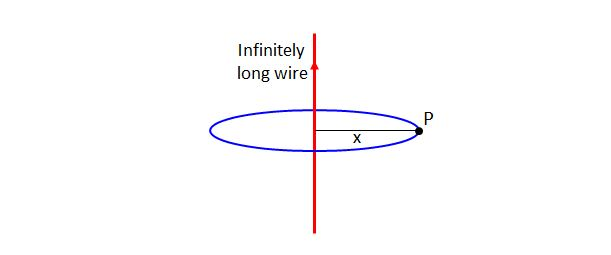# Electric field by straight wireSuppose that an infinitely long straight current-carrying wire has a uniform linear charge density $\lambda.$ Let this wire be on the $y$-axis of the $xy$-plane, and let $x > 0$ be the distance between the $y$-axis and a point $P$ on the $xy$-plane. What is the strength of the electric field at the point $P?$

Note: $\epsilon_0$ in the choices below denotes the electric constant.

×